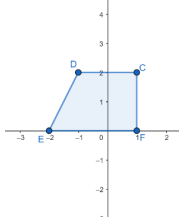# MA.912.GR.4.2Export Print
Identify three-dimensional objects generated by rotations of two-dimensional figures.

### Clarifications

Clarification 1: The axis of rotation must be within the same plane but outside of the given two-dimensional figure.
General Information
Subject Area: Mathematics (B.E.S.T.)
Strand: Geometric Reasoning
Status: State Board Approved

## Benchmark Instructional Guide

• Circle
• Cone
• Cylinder
• Prism
• Pyramid
• Rectangle
• Square
• Triangle

### Vertical Alignment

Previous Benchmarks

Next Benchmarks

### Purpose and Instructional Strategies

In grade 5, students identified three-dimensional figures. In Geometry, students visualize and discuss a three-dimensional figure as the result of a two-dimensional figure being rotated about an axis. In later courses, students will use rotations to find the volume of a three-dimensional figure (solid of revolution) using integrals. (MTR.5.1)
• Instruction begins with using a boundary line of a two-dimensional figure, including irregular figures, as the axis of the rotation, then move to an axis outside the two-dimensional figure.
• For example, when rotating a rectangle about one of its boundary lines, a cylinder will be generated. If the rectangle is rotated about a line that is parallel to a side and not touching the figure, a cylinder with a hole down its center (a washer) will be generated.
• For example, when rotating a right triangle about an axis containing one of the legs, a cone will be generated. When rotating a right triangle about the hypotenuse, a double cone will be generated.
• For enrichment purposes, include cases where an axis of symmetry is the axis of rotation; this will help make the connection of reflections to rotations about an axis.
• For example, if a non-square rectangle is rotated about a line of symmetry, then the three-dimensional figure that would be generated is a cylinder.
• Instruction includes exploring irregular shapes that can be rotated to generate vases or exploring the rotation of a sphere about an axis that is outside to generate a torus.
• Problem types include two-dimensional figures presented on the coordinate plane with vertical and horizontal axis of rotation. When presented on the coordinate plane, students can identify some attributes of the three-dimensional object generated by the rotation like the height or the radius.
• Instruction includes the use of models, such as straws and cardboard, or animations.

### Common Misconceptions or Errors

• Students may oversimplify when they try to visualize these rotations at first. To help address this, have students utilize physical models.

• Trapezoid DCFE is shown on the coordinate plane below.• Part A. If the trapezoid is extended to create right triangle EFB, what are the coordinates of point B
• Part B. If triangle EFB is rotated about line $x$ = 1, what figure will it generate?
• Part C. Determine the volume of the generated from Part B.
• Part D. If trapezoid DCFE is rotated about line $x$ = 1, describe the figure that is generated.
• Part E. Determine the volume of the generated from Part D.
• Part F. If trapezoid DCFE is rotated about line $x$ = 3, what figure will it generate?
• Part G. Determine the volume of the generated from Part F.

• Describe the figure that would be generated from the result of rotating a circle about a line outside the circle.

### Instructional Items

Instructional Item 1
•  Which real-world object could be used describe the figure generated by rotating a rectangle about a line that is parallel to a side but not touching the rectangle?
•  a. A doughnut
•  b. A piece of plastic tubing
•  c. An ice cream cone
•  d. A shoebox
•  e. An egg
*The strategies, tasks and items included in the B1G-M are examples and should not be considered comprehensive.

## Related Courses

This benchmark is part of these courses.
1200400: Foundational Skills in Mathematics 9-12 (Specifically in versions: 2014 - 2015, 2015 - 2022, 2022 and beyond (current))
1206310: Geometry (Specifically in versions: 2014 - 2015, 2015 - 2022, 2022 and beyond (current))
1206320: Geometry Honors (Specifically in versions: 2014 - 2015, 2015 - 2022, 2022 and beyond (current))
1206315: Geometry for Credit Recovery (Specifically in versions: 2014 - 2015, 2015 - 2022, 2022 and beyond (current))
7912065: Access Geometry (Specifically in versions: 2015 - 2022, 2022 and beyond (current))

## Related Access Points

Alternate version of this benchmark for students with significant cognitive disabilities.
MA.912.GR.4.AP.2: Identify a three-dimensional object generated by the rotation of a two-dimensional figure.

## Related Resources

Vetted resources educators can use to teach the concepts and skills in this benchmark.

## Formative Assessments

2D Rotations of Triangles:

Students are given the coordinates of the vertices of a right triangle and asked to describe the solid formed by rotating the triangle about a given axis.

Type: Formative Assessment

2D Rotations of Rectangles:

Students are given the coordinates of the vertices of a rectangle and asked to describe the solid formed by rotating the rectangle about a given axis.

Type: Formative Assessment

## MFAS Formative Assessments

2D Rotations of Rectangles:

Students are given the coordinates of the vertices of a rectangle and asked to describe the solid formed by rotating the rectangle about a given axis.

2D Rotations of Triangles:

Students are given the coordinates of the vertices of a right triangle and asked to describe the solid formed by rotating the triangle about a given axis.

## Student Resources

Vetted resources students can use to learn the concepts and skills in this benchmark.

## Parent Resources

Vetted resources caregivers can use to help students learn the concepts and skills in this benchmark.